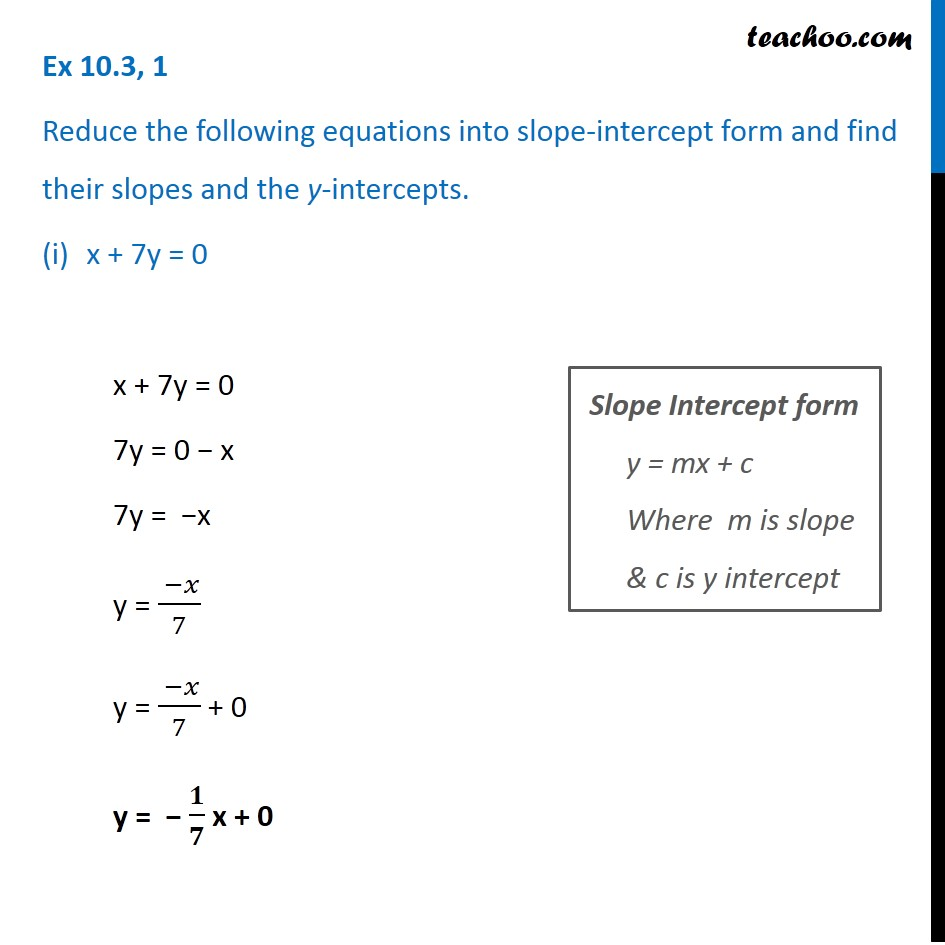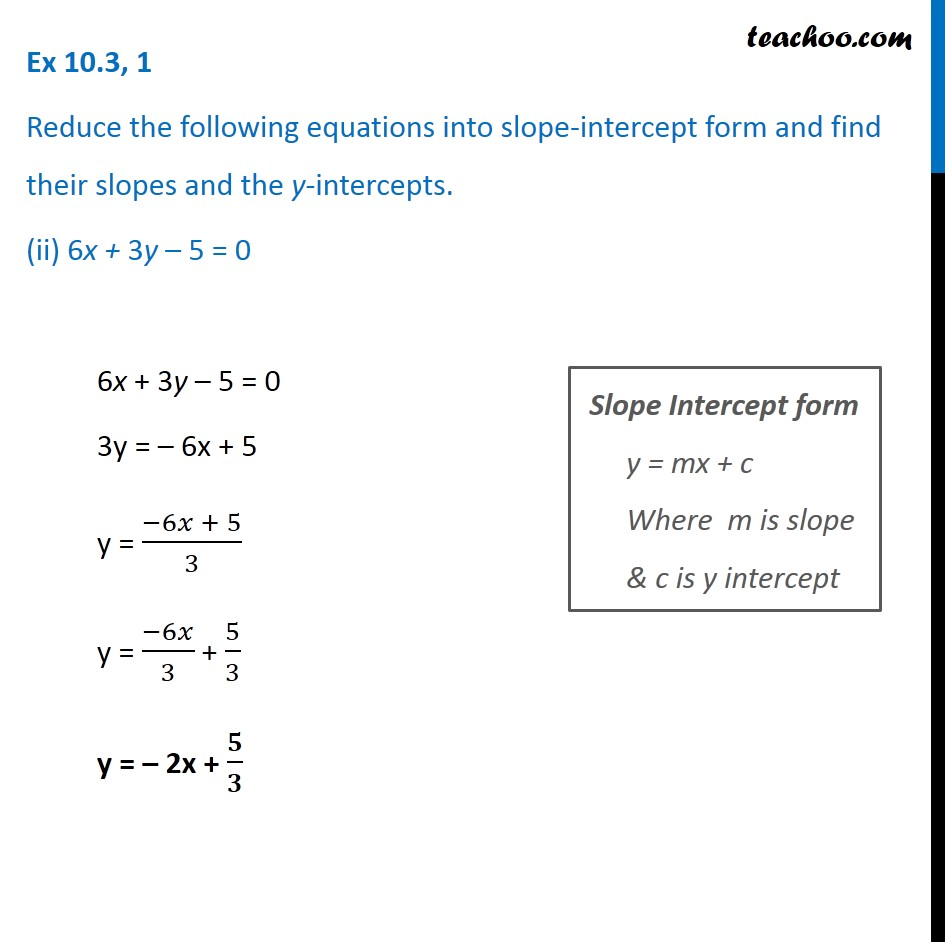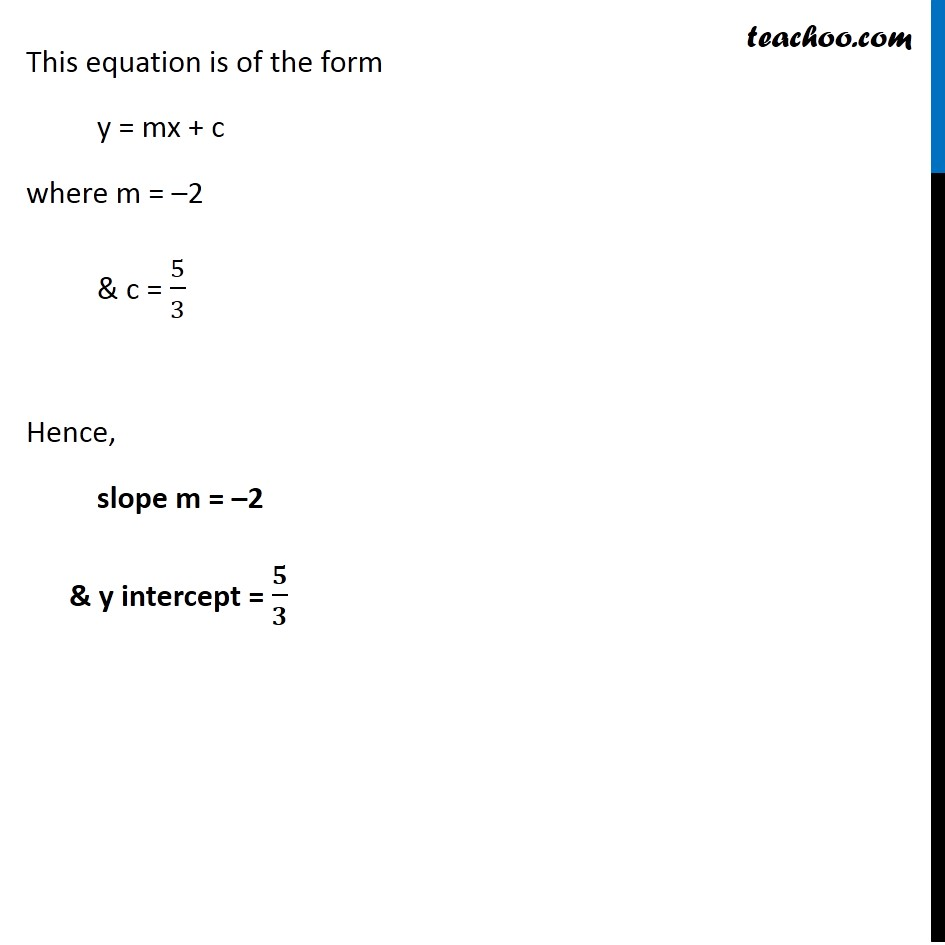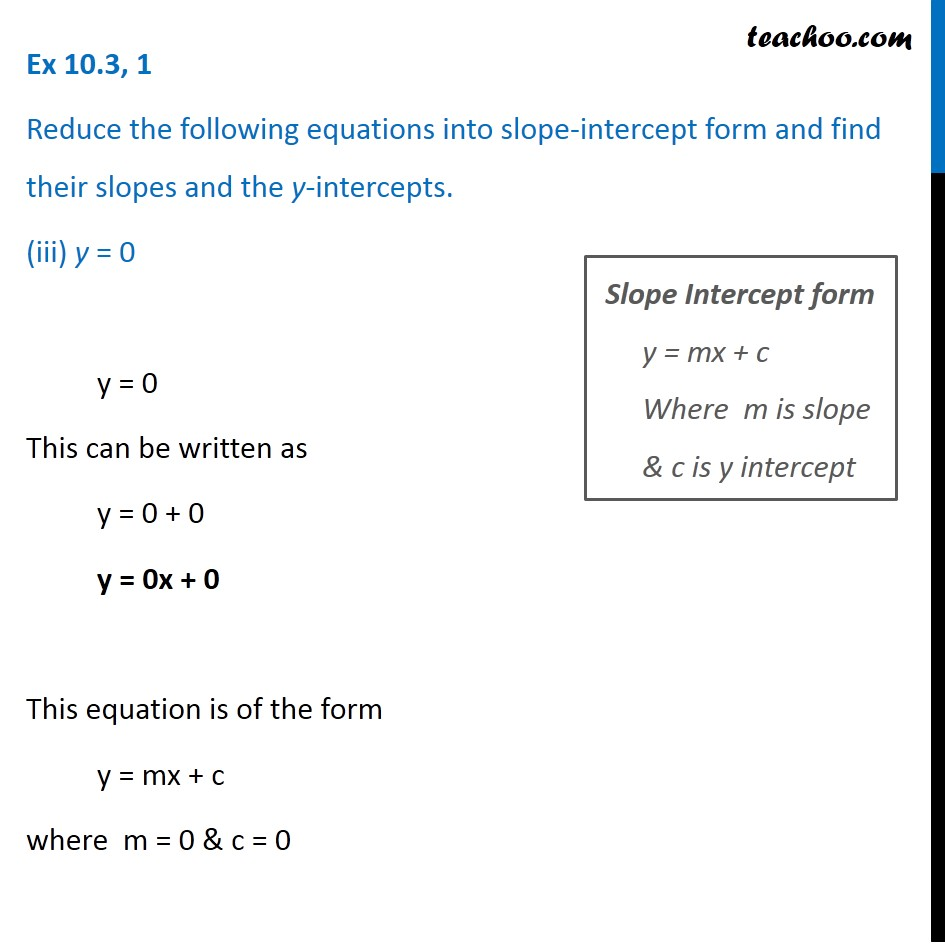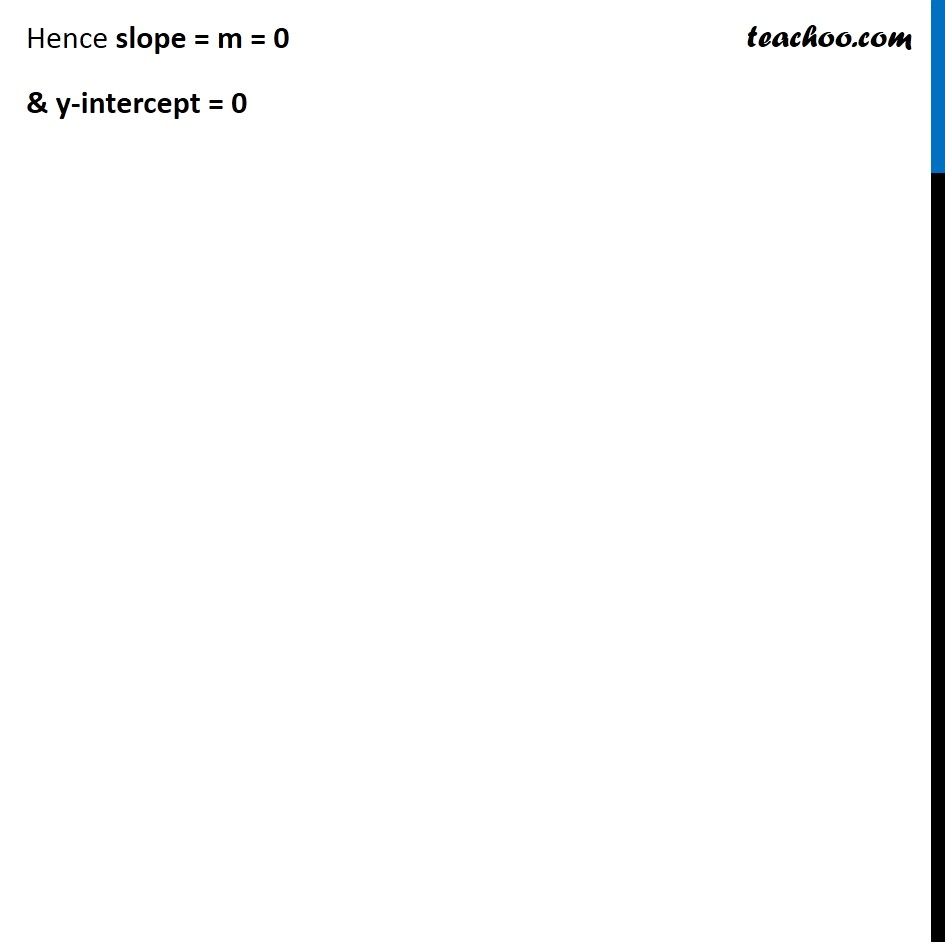Subscribe to our Youtube Channel - https://you.tube/teachoo

1. Chapter 10 Class 11 Straight Lines
2. Serial order wise
3. Ex 10.3

Transcript

Ex 10.3, 1 Reduce the following equations into slope-intercept form and find their slopes and the y-intercepts. x + 7y = 0 x + 7y = 0 7y = 0 − x 7y = −x y = ( −𝑥)/7 y = ( −𝑥)/7 + 0 y = − 𝟏/𝟕 x + 0 Ex 10.3, 1 Reduce the following equations into slope-intercept form and find their slopes and the y-intercepts. x + 7y = 0 x + 7y = 0 7y = 0 − x 7y = −x y = ( −𝑥)/7 y = ( −𝑥)/7 + 0 y = − 𝟏/𝟕 x + 0 Slope Intercept form y = mx + c Where m is slope & c is y intercept Ex 10.3, 1 Reduce the following equations into slope-intercept form and find their slopes and the y-intercepts. (ii) 6x + 3y – 5 = 0 6x + 3y – 5 = 0 3y = – 6x + 5 y = (−6𝑥 + 5)/3 y = (−6𝑥)/3 + 5/3 y = – 2x + 𝟓/𝟑 Slope Intercept form y = mx + c Where m is slope & c is y intercept This equation is of the form y = mx + c where m = –2 & c = 5/3 Hence, slope m = –2 & y intercept = 𝟓/𝟑 Ex 10.3, 1 Reduce the following equations into slope-intercept form and find their slopes and the y-intercepts. (iii) y = 0 y = 0 This can be written as y = 0 + 0 y = 0x + 0 This equation is of the form y = mx + c where m = 0 & c = 0 Slope Intercept form y = mx + c Where m is slope & c is y intercept Hence slope = m = 0 & y-intercept = 0

Ex 10.3